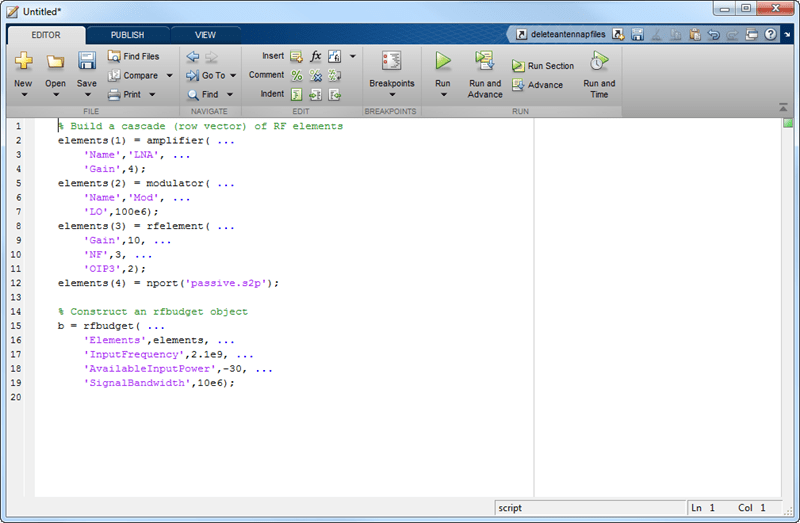# exportScript

Export MATLAB code that generates RF budget object

## Syntax

```exportScript(rfobj) ```

## Description

`exportScript(rfobj)` exports the MATLAB command-line code that generates an RF budget object. The script opens in an `Untitled*` window in the MATLAB editor.

## Input Arguments

expand all

RF budget analysis object, specified as a object handle.

## Examples

expand all

Create an RF budget object.

```a = amplifier('Name','LNA','Gain',4); m = modulator('ConverterType','Up','LO',100e6,'Name','Mod'); r = rfelement('Gain',10,'NF',3,'OIP3',2); n = nport('passive.s2p');```

Calculate the RF budget analysis.

`b = rfbudget([a m r n],2.1e9,-30,10e6);`

Export the analysis to a MATLAB script.

exportScript(b)